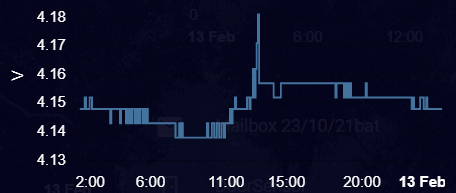# ESPHome Battery Level Sensor

I have a D1 mini connected to a 18650 battery. I would like to measure the voltage of the battery through the A0 pin on the D1 mini, but I’m not sure how to wire it. The A0 pin measures voltages between 0.0 - 1.0 volts and the 18650 outputs ~3.7 volts.

Can anyone point me in the right direction on how to wire the 18650 battery to the D1 mini and measure the battery voltage? I read this documentation: https://esphome.io/components/sensor/adc.html But I’m still not sure how to wire it all…

The d1 mini has a built in voltage divider so you can measure up to 3v3 on A0.

I also found this guide helpful:

https://ezcontents.org/esp8266-battery-level-meter

@bastero Juan one question on this one.

So the logic used is that A0 on D1 will output 3.3 V at max, if the measured voltage on A0 is say 2.97V then fair to say that battery level is 90% (2.97/3.3). Is this correct?

The maximum voltage that D1 can output is determined by its supply voltage i.e. 3.3V. (Vcc)

The input into A0 from the voltage divider is based on its supply voltage i.e. 3.7V and will always be a fraction of (Vbat), so yes your assumption is correct. Hope this clarifies.

Hi
The maximum output from the OP 18650 battery is 4.2V (for the nominal 3.7 V of what 18650 has).
The A0 pin is measuring the output of the battery not the output of the D1.
4.2 V would be enough to damage the D1 so you need to put a 220K Resistor between the battery and A0.
You will then need to multiply this by a factor to bring it up to 4.2 V when the 18650 if fully charged.
If you use ESPhome on the D1 you can put this factor in to the HTML for the ESPhome program to output directly as a sensor.
The 3.3 V output of the D1 shouldn’t change until battery is very low due to Lowdropout voltage regulator on the D1.
It looks like @bastero is using a voltage divider to drop the max 4.2 down to 3.3. Doesn’t sound like a good idea. I mean a terrible idea. Much better to use a LDL voltage regulator to bring battery voltage down to what the D1 uses. If you use a voltage divider to drop the voltage of the battery it will give a different voltage to power the D1 as the battery runs down. You want a constant voltage to the D1.

@bastero is not powering the d1 with the voltage divider. His circuit is shown.

I look closer and I stand corrected. Appologies @bastero. The idea of not using voltage divider to power D1 from a battery still is a good one do you agree? Voltage divider fine for measuring battery voltage.

I think you are quite right on that.

Out of interest, what resistor would you suggest between the + terminal of battery and A0 in the interests of not drawing much current from the battery but also not affecting the voltage reading much?

I would have thought the bigger the better. The math for the divider will be the same whether the resistors are large or small values, but the current draw (and battery wastage) will be smaller is the resistors are larger values.

Yes, I think ( as I’ve not got a background in electronics) that higher resistor values mean less current draw to the voltage measurement. I wonder then if you move up into mega ohm resistors then does the actual load (esp board) begin to affect the voltage reading to A0. I’m thinking of a reasonable compromise point that is generally accepted. In my setup I can detect about 5mv wobble which I have been putting down to the actual load. More than enough for my purpose. Just looking to learn more.Thanks for this all. Helped me a lot to develop my first battery level sensor, which I’ve added some details of here.

1 Like